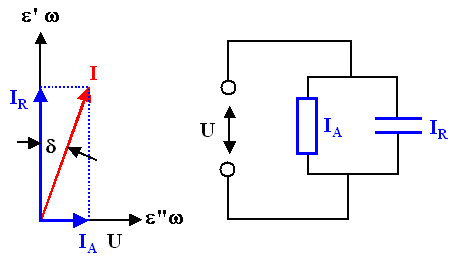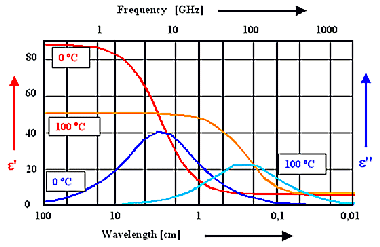### 3.4.2 Summary to: Dynamic Properties - Dielectric LossesThe frequency dependent current density j flowing through a dielectric is easily obtained. Þ
j(w)  =  dD
dt
=  e(w) · dE
dt
=  w · e'' · E(w)  +   i · w · e' · E(w)

in phase

out of phaseThe in-phase part generates active power and thus heats up the dielectric, the out-of-phase part just produces reactive powerThe power losses caused by a dielectric are thus directly proportional to the imaginary component of the dielectric function
LA
=

power turned
into heat
=  w · |e''| · E2The relation between active and reactive power is called "tangens Delta" (tg(d)); this is clear by looking at the usual pointer diagram of the currentLA
LR
:=  tg d  =   IA
IR
=   e''
e'The pointer diagram for an ideal dielectric s(w = 0) = 0can always be obtained form an (ideal) resistor R(w) in parallel to an (ideal) capacitor C(w).R(w) expresses the apparent conductivity sDK(w) of the dielectric, it follows that
sDK(w)  =  w · e''(w)For a real dielectric with a non-vanishing conductivity at zero (or small) frequencies, we now just add another resistor in parallel. This allows to express all conductivity effects of a real dielectric in the imaginary part of its (usually measured) dielectric function via
e''  =  stotal
wWe have no all materials covered with respect to their dielectric behavior - in principle even metals, but then resorting to a dielectric function would be overkill.A good example for using the dielectric function is "dirty" water with a not-too-small (ionic) conductivity, commonly encountered in food.The polarization mechanism is orientation polarization, we expect large imaginary parts of the dielectric function in the GHz region.It follows that food can be heated by microwave (ovens)!

 Questionaire Multiple Choice questions to all of 3.4

© H. Föll (Electronic Materials - Script)